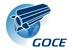#GOCE OWS data service

Goce WPS
Data
• gravitational potential V
Constant
• rSat = 6637655.2 is the radius of the geocentric sphere at mean satellite altitude in [m];
• gm = 3.986004415*10^14 is the product between the gravitational constant and the Earth mass [m^3/s^2];
• a = 6378137 ellipsoidal major radius [m];
• f = 1/298.257223563 ellipsoidal flattening;
• units for gravitational potential [m^2/s^2];
• units for second derivatives [mE] = [10^12/s^2].

#### Service

Interpolation in a user defined region

#### Output format data

GeoTIFF and ASCII GRID ESRI
Gemma WPS
Data
• Bathymetry
• Ice sheets
• Upper sediments
• Medium sediments
• Lower sediments
• Crust
• Upper mantle

#### Service

Interpolation in a user defined region or in a list of sparse points

#### Input format data

Sparse point: upload csv text file; separator char: | (pipe) => x|y
Example:
5.25|45.25
5.75|45.25
6.25|45.25
6.75|45.25

#### Output format data

If required interpolation in a region: GeoTIFF and ASCII GRID ESRI
If required interpolation in a list of sparse points: CSV text file